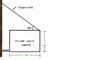# Solving Force on a Hanging Sign

• fball558

#### fball558

hanging sign forces??

## Homework Statement

PIC IS ATTACHED

A businessman is hanging a sign outside his store in the
fashion shown in the figure below. The sign has a mass of
50 kg. For a sign of this mass, what is the tension in the
wire? The sign is rigidly attached to the wall at the lower
left corner.

A. 346 N
B. 260 N
C. 490 N
D. 87 N
E. 173 N

## The Attempt at a Solution

i am stuck. only thing i though of was find mg then find the sin of the angle
so i did mg sin (45)
490 sin (45) got 370.
which is not even close to letter E (which is the right answer)

#### Attachments

•sign.jpg
13.3 KB · Views: 553

If it's rigidly attached as it says in the question - then the tension in the wire is zero.
ie the sign would stay there without the wire

Normally in these question you assume the weight acts at the centre of the sign and take moments about the point that it joins the wall (assumed to pivot) to find the upward force at the outside end

The question is flawed.
Answer E is obtained if you assume the sign
can pivot freely about the bottom left corner.of the sign.
(hardly "attached rigidly"!)

Remembor the pivot can exert a force on the sign, but no torque.

David

The question is flawed.
Answer E is obtained if you assume the sign
can pivot freely about the bottom left corner.of the sign.
(hardly "attached rigidly"!)

Remembor the pivot can exert a force on the sign, but no torque.

David

so if we assume the sign can pivot, how would you go about getting answer E??